## 春招已近，这份GitHub万星的ML算法面试大全请收下

2 月 22 日 全球人工智能• 项目地址：https://github.com/imhuay/Algorithm_Interview_Notes-Chinese• 信息论

• 逻辑斯蒂回归

• 支持向量机

• 决策树

• 集成学习

• 梯度提升决策树 GBDT

• 随机森林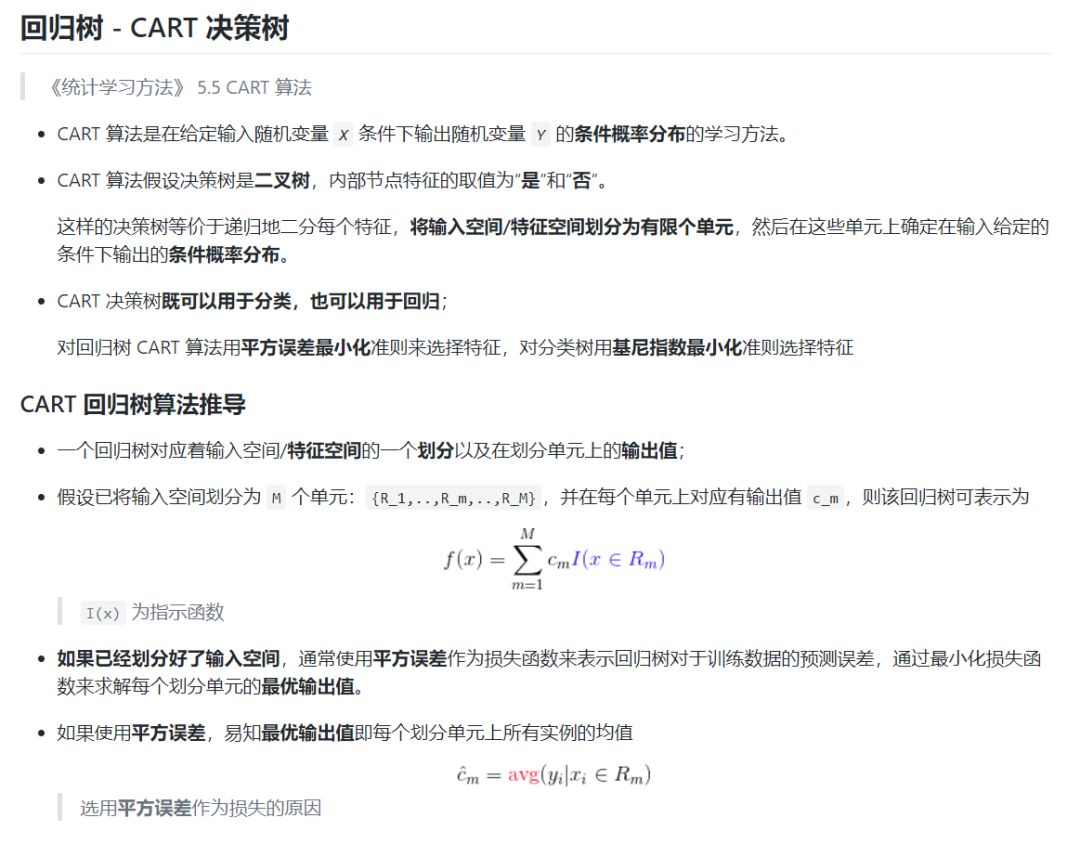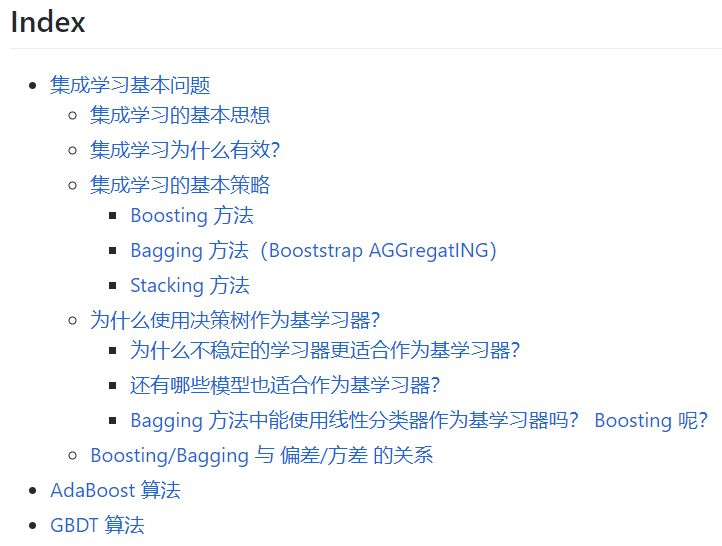• 深度学习基础

• 深度学习实践

• CNN 专题

• RNN 专题

• 优化算法专题

• 序列建模专题

• 《Deep Learning》整理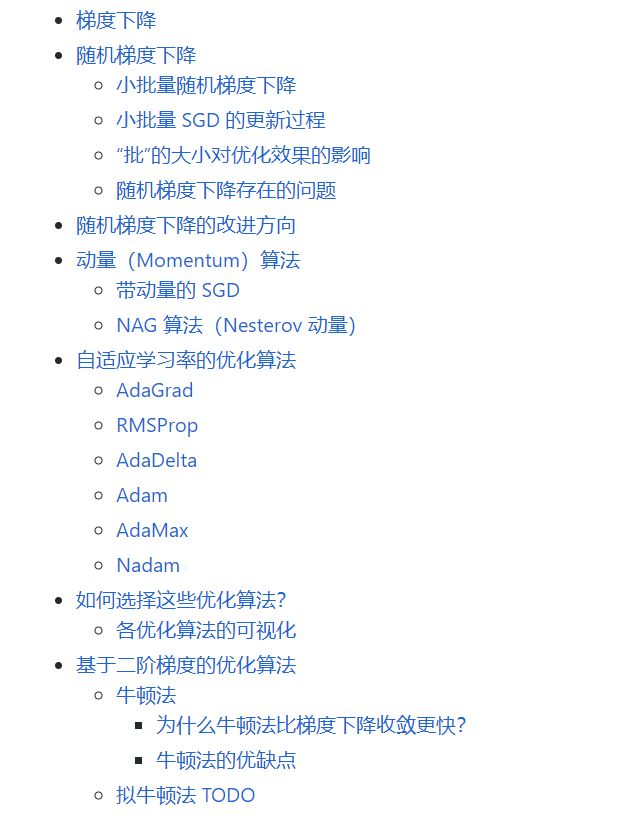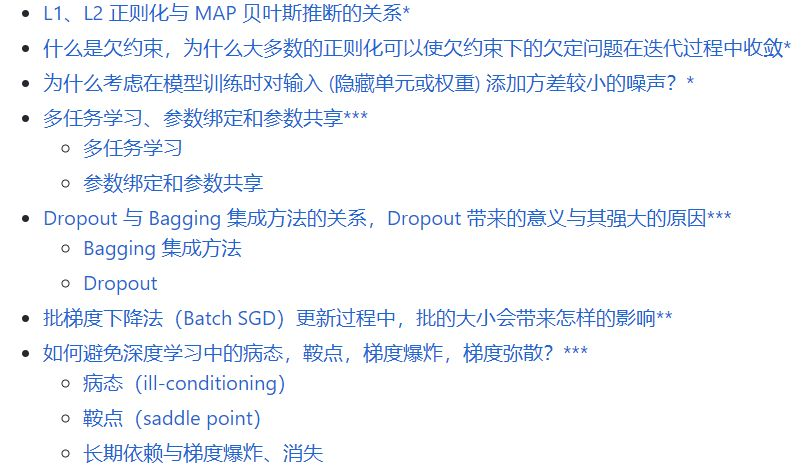• 自然语言处理基础

• NLP 发展趋势

• 词嵌入专题

• 句嵌入专题

• 多模态专题

• 视觉问答综述

• 深度理解查询

NLP 很多知识点其实都不算基础内容，这需要根据我们自己学习的领域收集复习内容。不过像 NLP 基础或词嵌入等知识点，项目作者介绍得很详细，它们也是 NLP 面试必备知识。

• 概率论

• 微积分本质

• 深度学习核心

• 字符串

• 数据结构

• 高级数据结构

• 动态规划

• 双指针

• 区间问题

• 排列组合

• 数学问题

• Shuffle、采样、随机数

• 大数运算

• 海量数据处理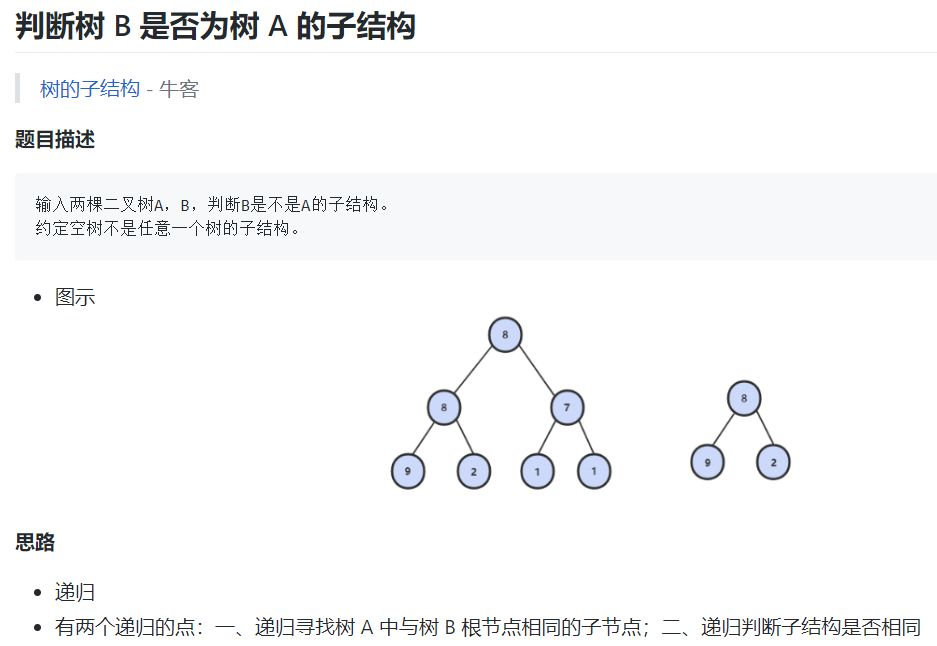``class Solution {public:    bool HasSubtree(TreeNode* p1, TreeNode* p2) {        if (p1 == nullptr || p2 == nullptr)  // 约定空树不是任意一个树的子结构            return false;        return isSubTree(p1, p2)    // 判断子结构是否相同            || HasSubtree(p1->left, p2)      // 递归寻找树 A 中与树 B 根节点相同的子节点            || HasSubtree(p1->right, p2);    }    bool isSubTree(TreeNode* p1, TreeNode* p2) {        if (p2 == nullptr) return true;        // 注意这两个判断的顺序        if (p1 == nullptr) return false;        if (p1->val == p2->val)            return isSubTree(p1->left, p2->left)    // 递归判断左右子树                && isSubTree(p1->right, p2->right);        else            return false;    }};``

``// 示例 1int a, b;while (scanf("%d %d", &a, &b) != EOF && (a != 0 && b != 0)) {    // ...}// 或者while (scanf("%d %d", &a, &b) != EOF && (a || b)) {    // ...}// 示例 2int n;while (scanf("%d", &n) != EOF && n != 0) {    // ...}``

``// 示例 1int a, b;while (cin >> a >> b) {    if (a == 0 && b == 0)        break;    // ...}// 示例 2int n;while (cin >> n && n != 0) {    // ...}``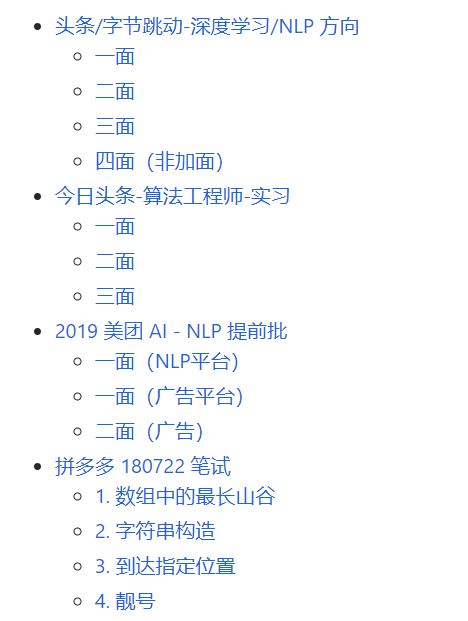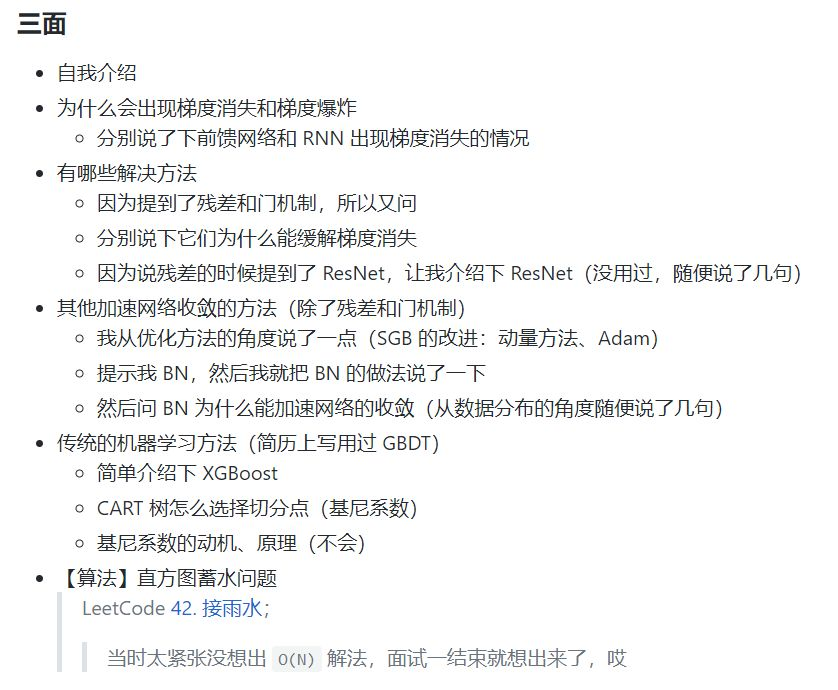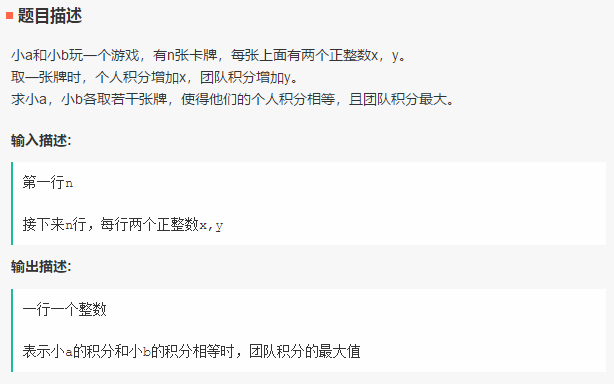``# 输入处理n = int(input())x, y = [], []for i in range(n):    _x, _y = list(map(int, input().split()))    x.append(_x)    y.append(_y)xy = list(zip(x, y))xy = sorted(xy, key=lambda t: t)ret = 0if sum(x) % 2 == 0:  # 如果所有 x 的和为偶数    print(sum(y))    # 直接输出所有 y 的和else:    for i in range(len(xy)):        if xy[i] % 2 == 1:  # 去掉 x 中为奇数的那一项            ret = sum([xy[j] for j in range(len(xy)) if j != i])            print(ret)            break``Attention Model has now become an important concept in neural networks that has been researched within diverse application domains. This survey provides a structured and comprehensive overview of the developments in modeling attention. In particular, we propose a taxonomy which groups existing techniques into coherent categories. We review the different neural architectures in which attention has been incorporated, and also show how attention improves interpretability of neural models. Finally, we discuss some applications in which modeling attention has a significant impact. We hope this survey will provide a succinct introduction to attention models and guide practitioners while developing approaches for their applications.We introduce an adversarial learning framework, which we named KBGAN, to improve the performances of a wide range of existing knowledge graph embedding models. Because knowledge graph datasets typically only contain positive facts, sampling useful negative training examples is a non-trivial task. Replacing the head or tail entity of a fact with a uniformly randomly selected entity is a conventional method for generating negative facts used by many previous works, but the majority of negative facts generated in this way can be easily discriminated from positive facts, and will contribute little towards the training. Inspired by generative adversarial networks (GANs), we use one knowledge graph embedding model as a negative sample generator to assist the training of our desired model, which acts as the discriminator in GANs. The objective of the generator is to generate difficult negative samples that can maximize their likeliness determined by the discriminator, while the discriminator minimizes its training loss. This framework is independent of the concrete form of generator and discriminator, and therefore can utilize a wide variety of knowledge graph embedding models as its building blocks. In experiments, we adversarially train two translation-based models, TransE and TransD, each with assistance from one of the two probability-based models, DistMult and ComplEx. We evaluate the performances of KBGAN on the link prediction task, using three knowledge base completion datasets: FB15k-237, WN18 and WN18RR. Experimental results show that adversarial training substantially improves the performances of target embedding models under various settings.Top